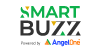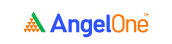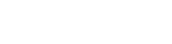# How Valuations are Important to derive Market ratiosIf you remember, in module 4 of Smart Money, we talked about a lot of key ratios and metrics. Those key balance sheet and P&L ratios served only one purpose - to enable you to better understand the fundamentals and the financial situation of the company.

In this chapter, we’ll again be looking at several key ratios. However, unlike the others, these ratios serve two purposes.

1. They enable you to determine the value of the stock of a company.
2. They enable you to compare the stock valuation of multiple companies.

These key ratios that we’re about to see are also known as ‘valuation ratios’ since they help you determine the valuation of a company. These ratios, when used right, can give you some much-needed information on whether the stock of a company is overvalued or undervalued.

Since we’ve already extensively researched the other key ratios of Hindustan Unilever Limited in the previous module, we’ll take up HUL once again for our valuation ratio calculations as well. So, without any further ado, let’s jump right in, starting with the price to earnings (P/E) ratio.

## 1. Price to earnings (P/E) ratio

One of the most widely used ratios, the P/E ratio is a key part of valuation ratios. It is used to establish a relationship between the current market price of a company’s stock to its earnings per share. Calculating the P/E ratio is pretty simple. Here’s the formula.

 P/E ratio = current market price per share ÷ earnings per share

A high P/E ratio could mean that the company’s stock is overvalued, whereas a low P/E ratio could mean that a company’s stock is undervalued. Let’s apply this formula and calculate the P/E ratio of Hindustan Unilever Limited for the current year.

Current market price of HUL (as on March 31, 2020): Rs. 2,298.50We can find the earnings per share of HUL for the year 2019-2020 from the annual report of the company, under the notes to financial statements.

Earnings per share of HUL (for the year 2019-2020): Rs. 31.12So, here’s the P/E ratio of HUL as on March 31, 2020.

P/E ratio of HUL (as on March 31, 2020) = 73.86

(Rs. 2,298.50 ÷ Rs. 31.12)

## 2. Price to sales (P/S) ratio

The price to sales ratio helps establish the relationship between the current share price of a company and its sales. It gives you a fair idea of how much the company’s stock is valued with respect to a rupee’s worth of sales. You can calculate this ratio using this formula.

 Price to sales (P/S) ratio = market capitalisation ÷ total revenue

Where,

Market capitalisation = current share price x total number of shares

Let’s put this in context with respect to HUL.The total number of outstanding equity shares of the company can be found under the ‘notes to financial statements’ section of HUL’s annual report.This gives us the market cap as calculated below.

Market capitalisation (as on March 31, 2020) = Rs. 4,96,476 crores

(Rs. 2,298.50 x 216 crore shares)

With respect to the total revenue of HUL, it can be found in the company’s P&L statement itself: Rs. 39, 518 crores.

Price to sales (P/S) ratio of HUL (as on 31st March, 2020) = 12.56

(Rs. 4,96,476 crores ÷ Rs. 39,518 crores)A P/S ratio of 12.56 indicates that for every rupee’s worth of sales, HUL’s stock price is Rs. 12.56 times higher.

## 3. Price to book (P/B) ratio

The price to book value ratio is used to establish a relationship between the current market price of a company’s stock to its book value per share.

A high P/B ratio could mean that the company’s stock is overvalued, whereas a low P/B ratio could mean that a company’s stock is undervalued. Calculating the P/B ratio is simple. Here’s the formula.

 P/B ratio = current market price per share ÷ book value per share

Where the book value per share = total equity ÷ total number of shares

We’ll apply this formula to calculate the P/B ratio of Hindustan Unilever Limited for the year 2019-2020. We can get the value of total equity from the balance sheet of HUL.The total number of outstanding equity shares of the company can be found under the ‘notes to financial statements’ section of HUL’s annual report.The book value per share of HUL for the year 2019-2020 = Rs. 37.18

(Rs.8,031 crores ÷ Rs. 216 crores)

Now that we’ve calculated the book value per share, let’s move on to calculating the P/B ratio of HUL as on 31st March, 2020.

The current market price of Hindustan Unilever Limited as on 31st March, 2020 is as follows.Price to book (P/B) ratio of HUL (as on 31st March, 2020) = 61.82

(Rs. 2,298.50 ÷ Rs. 37.18)

A P/B ratio of 61.82 indicates that the market price of each share of HUL is currently trading at 61.82 times of its book value per share.

## 4. Price to cash flow (P/CF) ratio

The price to cash flow ratio establishes the relationship between the current market price of a company’s shares and its operating cash flow per share. A low P/CF ratio indicates that the stock of a company is undervalued, whereas a high P/CF ratio is indicative of overvaluation.

 P/CF ratio = current market price per share ÷ operating cash flow per share

Where the operating cash flow per share = operating cash flow ÷ total number of shares

Let’s apply this formula to Hindustan Unilever Limited and calculate the price to cash flow ratio of the company for the year 2019-2020.

The value of operating cash flow for the year 2019-2020 can be found in the cash flow statement in the annual report.The operating cash flow per share of HUL (for the year 2019-2020) = Rs. 33.82

(Rs. 7,305 crores ÷ 216 crores)

Now that we’ve calculated the operating cash flow per share, let’s move on to the calculation of the P/CL ratio of HUL as on 31st March, 2020.

The current market price of Hindustan Unilever Limited as on 31st March, 2020 is as follows.Price to cash flow (P/CL) ratio of HUL (as on 31st March, 2020) = 67.96

(Rs. 2,298.50 ÷ Rs. 33.82)

A P/CL ratio of 67.96 indicates that the market price of each share of HUL is currently trading at 67.96 times of its operating cash flow per share.

## 5. Enterprise market value (EMV)

The enterprise market value is an easy and quick way to assess the value of a company. It can help determine a company’s total value and is typically used during potential takeovers of companies.

 Enterprise market value (EMV) = market capitalisation + total debt - cash

Let’s apply this formula for HUL to determine its enterprise market value.

We already have the value of market capitalisation of HUL from our earlier calculations. The total debt (both current and non-current debt) as well as the value of cash held by the company can be found in the balance sheet.The enterprise market value of HUL (for the year 2019-2020) = Rs. 5,03,030 crores

(Rs. 4,96,476 crores + Rs. 11,571 crores - Rs. 5,017 crores)

### Wrapping up

Okay, so now that you’ve learnt how to use these ratios for determining the value of a company, what do you do with this information? The values put out by these ratios might not mean much when read in a stand alone manner. To get a better sense of a company and its value, it is advisable to compare these values with those of comparatively similar companies. This way, you can easily determine whether the company’s stock is worth the price it is currently trading at.

### A quick recap

• Valuation ratios help you determine the valuation of a company. These ratios, when used right, can give you some much-needed information on whether the stock of a company is overvalued or undervalued.
• The P/E ratio is a key part of valuation ratios. It is used to establish a relationship between the current market price of a company’s stock to its earnings per share.
• P/E ratio = current market price per share ÷ earnings per share
• The price to sales ratio helps establish the relationship between the current share price of a company and its sales. It gives you a fair idea of how much the company’s stock is valued with respect to a rupee’s worth of sales.
• Price to sales (P/S) ratio = market capitalisation ÷ total revenue
• The price to book value ratio is used to establish a relationship between the current market price of a company’s stock to its book value per share.
• P/B ratio = current market price per share ÷ book value per share
• The price to cash flow ratio establishes the relationship between the current market price of a company’s shares and its operating cash flow per share. A low P/CF ratio indicates that the stock of a company is undervalued, whereas a high P/CF ratio is indicative of overvaluation.
• P/CF ratio = current market price per share ÷ operating cash flow per share
• The enterprise market value is an easy and quick way to assess the value of a company. It can help determine a company’s total value and is typically used during potential takeovers of companies.
• Enterprise market value (EMV) = market capitalisation + total debt - cashTake the quiz for this chapter & mark it complete.

How would you rate this chapter?

YASH JAIN

09 May 2021, 12:41 PM

Thank You So Much It's Help Me Lot..

Get Information Mindfulness!

Catch-up With Market

News in 60 Seconds.The perfect starter to begin and stay tuned with your learning journey anytime and anywhere.